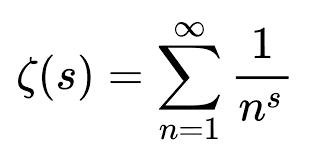Staff EmeritusEuler’s amazing identity
The mathematician Leonard Euler developed some surprising mathematical formulas involving the number ##\pi##. The most famous equation is ##e^{i \pi} = -1##, which is one of the most important equations in modern mathematics, but unfortunately, it wasn’t invented by Euler.Something that is original with Euler is this amazing identity:
Equation 1: ##1 + 1/4 + 1/9 + 1/16 + … = \pi^2/6##
This is one instance of an important function called the Riemann Zeta function, ##\zeta(s)##, which in the case where ##s > 1## is defined by:
Equation 2: ##\zeta(s) = \sum_{j=1}^\infty \dfrac{1}{j^s}##
So Euler’s identity can be written as:
Equation 3: ##\zeta(2) = \frac{\pi^2}{6}##
This post is an attempt to show how you can derive that result, and related results, using facts about trigonometry, complex numbers, and the Fourier series.
Some related functions defined...

#### Attachments

Last edited by a moderator:
•WWGD, Paul Colby and Greg Bernhardt

## Answers and Replies

SSequence
Just below the heading "Equation-7". Also same identity below the heading "Equation-9" and before "Sum-1".
##F(x)= \sum_{j=-\infty}^{\infty} e^{ijx}=\sum_{j=-\infty}^{-1} e^{ijx}+1+\sum_{j=0}^{\infty} e^{ijx} ##

Shouldn't it be(?):
##F(x)= \sum_{j=-\infty}^{\infty} e^{ijx}=\sum_{j=-\infty}^{-1} e^{ijx}+1+\sum_{j=1}^{\infty} e^{ijx} ##

•